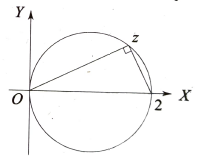Geometry of complex numbers
Question

# If z lies on the circle $\left|\mathrm{z}-1\right|=1$ then $\frac{\mathrm{z}-2}{\mathrm{z}}$ equals

Moderate
Solution

##Note that $\left|\mathrm{z}-1\right|=1$represents a circle with the segment joining z = 0 and z = 2 +0i as a diameter.  If z lies on the circle, then $\frac{\mathrm{z}-2}{\mathrm{z}-0}$  is purely imaginary. ( since the angle at z is $\frac{\mathrm{\pi }}{2}$)

Get Instant Solutions